International Journal of Fluid Mechanics & Thermal Sciences
Volume 2, Issue 4, December 2016, Pages: 37-46

Experimental Investigation of Pulsating Turbulent Flow Through Diffusers

Masaru Sumida

Department of Mechanical Engineering, Faculty of Engineering, Kindai University, Higashi-Hiroshima, Japan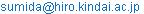Masaru Sumida. Experimental Investigation of Pulsating Turbulent Flow Through Diffusers. International Journal of Fluid Mechanics & Thermal Sciences. Vol. 2, No. 4, 2016, pp. 37-46. doi: 10.11648/j.ijfmts.20160204.12

Received: November 29, 2016; Accepted: December 26, 2016; Published: January 16, 2017

Abstract: This paper presents the results of an experimental study on a pulsating turbulent flow through conical diffusers with total divergence angles (2θ) of 12°, 16°, and 24°, whose inlet and exit were connected to long straight pipes. To examine the effects of the divergence angle and the nondimensional frequency on flow characteristics, experiments were systematically conducted using a hot-wire anemometry and a pressure transducer. Moreover, the pressure rise between the inlet and the exit of the diffuser was analyzed approximately under the assumption of a quasi-steady flow and expressed in the form of simple empirical equations in terms of the time-mean value, the amplitude, and the phase difference from the flow rate variation. The expressions are in good agreement with the experimental results and very useful in practice. With the increase in the Womersley number, α, and 2θ, the sinusoidal change in the phase-averaged velocity, W, with time becomes distorted, and the W distributions show a more complicated behavior. For the flow at α=10 in the diffusers with large 2θ, the distributions of W are depressed on the diffuser axis. In contrast, for the flow at α=20, W has a protruding distribution on the diffuser axis.

Keywords: Pulsating Flow, Diffuser, Velocity Distribution, Pressure Distribution, Womersley Number, Divergence Angle

Contents

1. Introduction

The objective of this study is experimentally investigating the characteristics of an unsteady turbulent flow in diffusers. To this end, we consider a volume-cycled pulsating flow as the subject of the flow problem and carry out pressure and velocity measurements for conical diffusers with divergence angles (2θ) of 12°, 16°, and 24°. We examine the flow behaviors of the pressure and velocity distributions and clarify the effects of the divergence angle and the unsteady flow parameters on them.

Flow in diffusers occurs in the expansion passages in fluid machinery equipment and are also assumed to occur in cascades between the blades of pumps and compressors. Thus, the flow in diffusers is an important flow problem in fluid engineering. Therefore, they have been actively studied for over half a century [1, 2]. In the studies performed until the 1980s, the recovery efficiency of the pressure, the flow loss in the diffuser geometries, and the effects of the inlet conditions on their characteristics were examined comprehensively. However, in the studies, the inlet flow to the diffusers was steady.

The above is a general view of the research situation in fluid engineering for conventional industry. Moreover, in the 2000s, the unsteady flow through a divergent passage and a diffuser attracted increasing interest for applications in new fields such as automotive catalysts, microdiffusers, and vocal folds.

The flow distribution across a catalytic converter is very important to improve its effectiveness. Benjamin et al. , using a hot-wire anemometry, measured the distribution of a pulsating airflow within a system consisting of a section with divergence angles, 60° and 180°, and examined the effect of the pulsation on the flow uniformity. Moreover, King and Smith  and Mat Yamin et al.  reported on the uniformity of distributions of the flow in a wide-angled planar diffuser and downstream of catalyst monoliths, where measurements were carried out under engine operating conditions using a hot-wire anemometry and a cycle-resolved particle image velocimetry, respectively. The separation in an oscillating flow for a geometry with an adverse pressure gradient was studied, and also the separation in the accelerative phase was compared with that in the decelerative phase.

There is a rapidly growing interest for the analysis of the flow in microdiffusers to optimize the performance of devices, and recent advances in the analysis of flow were surveyed by Nabavi . Most of the studies on unsteady flows through microdiffusers have been carried out for planar microdiffusers [12, 13]. However, there have been only a few studies [14, 15] on conical microdiffusers, based on numerical analysis and experiments. In these studies, the flow rectification performance of conical diffusers was examined, but the investigation was limited to a laminar flow at a very low Reynolds number owing to the characteristics of the microdevice. In addition, the inlets and exits of microdiffusers are manufactured with various shapes and ports, depending on the application, in contrast to those with straight tubes. Therefore, no general findings have been reported.

Research on a pulsating flow in a diffuser has been carried out to ascertain the behavior of the physiologically driven flow in the larynx. Erath and Plesniak [16, 17] investigated the pulsating flow through a one-sided diffuser and a divergent vocal-fold model under corresponding life-size conditions.

Thus, there is a growing necessity for research on pulsating flow in conical diffusers. However, even in the studies of Benjamin et al.  and Wang and coworkers [14, 15] on conical diffusers, the velocity distributions for the diffusers were not provided, except for those at the inlet and exit planes. Therefore, studies that are more comprehensive are required. With this background, Sumida  carried out preliminary experiments on a pulsating turbulent flow in a conical diffuser with a divergence angle (2θ) of 12°. It was found that the distributions of the pressure and velocity exhibit complicated behaviors, which are different from those in a steady flow. Note that a divergence angle (2θ) of 6-8°, or 12° is known to be the optimum value for conical diffusers in internal flow systems . In this case, the length in the axial direction of the diffuser becomes large. However, considering the need to fit the diffuser into a pipeline in practical use, the divergence angle is often increased to reduce the length of the diffuser.

In this study, on the basis of the findings of the preceding report , the pulsating-flow problem is investigated for conical diffusers with larger angles of divergence. The velocity is measured by a hot-wire anemometry, and the distributions of the phase-averaged velocity are examined. Moreover, the time dependence of the pressure difference between the inlet and the exit of the diffusers is obtained experimentally and analyzed approximately under the assumption of a quasi-steady flow. Thereafter, the effects of the pulsation frequency and divergence angle on the flow characteristics are clarified in comparison with those in a steady flow.

2. Experimental Apparatus and Procedure

2.1. Experimental Apparatus

A schematic diagram of the experimental apparatus employed in this experiment is shown in Fig. 1. The system consists of a pulsating-flow generator, a test diffuser, and devices for measuring velocity and pressure. The working fluid was air at room temperature. The volume-cycled pulsating flow was composed of a steady flow and an oscillating flow. The steady flow, i.e., a time-mean flow, was supplied through a surge tank by a 750 W suction blower, which ensured that the flow rate was independent of the superposed oscillations and the test diffusers. The volume-cycled oscillating flow, on the other hand, was supplied by a pneumatic piston cylinder reciprocating with a stepping motor. The diameter of the cylinder was 300 mm, and the stroke of the piston could be varied from 0 to 1000 mm. The cycle and amplitude of the oscillating flow were controlled and set using a personal computer (PC). Thus, the desired pulsating flow rate in the sinusoidal waveform was effectuated in the test diffuser, and the periodic flow rate, Q,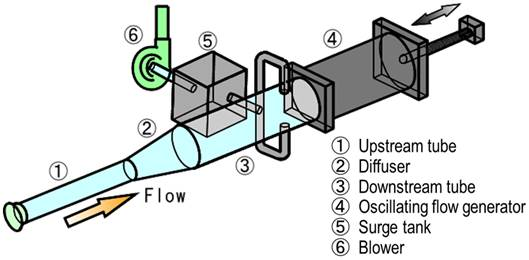Figure 1. Schematic diagram of the experimental apparatus.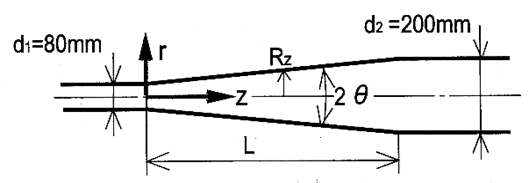Figure 2. Conical diffusers and coordinate system.

Table 1. Dimensions of the test diffusers.

 Diffuser Divergence angle 2θ,° Length L, mm (L/d1) I 12 568 (7.1) II 16 427 (5.34) III 24 282 (3.53)

is expressed as

Q = Qta + Qos sin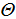(1)

Here,(= ωt) is the phase angle; ω is the angular frequency of pulsation, and t is the time. Moreover, subscripts, ta and os, indicate the time mean and amplitude, respectively.

Three conical diffusers were employed in the present study, as shown in Fig. 2, together with the coordinate system. The origin of the coordinate system is the diffuser axis at the inlet of the diffuser; z is the length measured along the diffuser axis from the diffuser inlet; Rz is the distance between the diffuser axis and the wall along the r-axis. The divergence angle, 2θ, and the diffuser axial length, L, are given in Table 1. The diffusers were constructed from three to five transparent acrylic blocks, which were accurately manufactured by a machining process. The blocks were connected via a slip ring, which had static pressure holes of 0.8 mm diameter spaced 90° apart and a small hole to insert a hot-wire probe. The diameters at the inlet and exit of the diffusers were d1=80 mm and d2 =200 mm, respectively, with an area ratio of 6.25, calculated using m [= (d2 /d1)2], where subscripts 1 and 2 denote the values in the upstream and downstream tubes, respectively. The divergence angles (2θ) of the diffusers I to III were 12°, 16°, and 24°, respectively, which give an ideal pressure coefficient of the diffuser, Cp,th = 0.97, which is written as Cp,th =1-m-2. The straight transparent glass tubes with lengths of 3700 mm (=46.3d1) and 4200 mm (=21d2) were connected to the inlet and the exit of the diffuser, respectively.

2.2. Measurement Procedures

Measurements were carried out for static pressure, p, on the wall and velocity, w, in the axial direction. The wall static pressure was obtained using a diffusive-type semiconductor pressure transducer (Toyoda MFG, DD102-0.1F). The measuring positions were set at 9 to 11 streamwise stations between z= -22.1d1 in the upstream straight tube and z =21.9d1 in the downstream one. In the experiment, the instantaneous pressure, p, at each position was concretely obtained as the pressure difference, p-pref, from the reference pressure, pref, at z/d1= -2 in the upstream tube. Furthermore, the phase-averaged value, P-Pref, was calculated, and its distribution in the z-direction was examined.

On the other hand, the axial-velocity measurements were performed using a constant-temperature hot-wire anemometry (Kanomax, System 7112) with a temperature compensation circuit. An I-type probe with a tungsten wire of 5 mm diameter and an active length of 1 mm was used. The probe was calibrated using a circular nozzle with an area of 1960 mm2. The hot-wire probe was mounted on a traversing device controlled by a PC and aligned at a prescribed position with an accuracy within 0.05 mm. The velocity, w, was obtained at six, five, and four stations for the diffusers from I to III, respectively. In addition, w was measured at z/d1= -2 in the upstream tube and at a section 2.5d1 downstream from the diffuser exit. The voltage output from the anemometry was sampled using the PC synchronously with a time-marker signal that indicates the position of the piston. The data at each measuring point were obtained for 200-500 pulsation cycles and 360 data per cycle were sampled at equal time intervals.

From the collected data, the phase-averaged velocity, W, and turbulence intensity, w’, were obtained. However, it is known that the velocities in the pulsating turbulent flow will have very complex distributions. In this study, we investigate the phase-averaged velocity W. To obtain W at each phase, the data of the cycles were superimposed and ensemble phase-averaged velocity into one cycle. This procedure was also applied to the phase-averaged value, P, of the wall static pressure, p.

In the pulsating-flow measurements, it is important to consider the effect of averaging over the pulsation cycles on W and w’. To determine this effect, preliminary measurements were carried out at intermediate stations of each diffuser and at z= -2d1 in the upstream tube. The obtained results confirmed that averaging over 200 cycles has no effect on W. The scatter in the results increased slightly with increasing divergence angle, but remained less than 4% for W. However, in the hot-wire measurement, we cannot easily accurately determine the direction of the flow at a position and time when the ratio of the radial component to the axial component of the velocity is large. For such a case, a certain amount of error is unavoidable , particularly near the wall. Furthermore, we also verified that the flow properties were symmetric with respect to the diffuser axis. In addition, the time-dependent flow rate, which was calculated by integrating the velocity measured at z/d1= -2 over the cross section, was confirmed to be highly sinusoidal and in good agreement with Eq. (1). That is, the difference between the obtained and prescribed flow rates was less than approximately 4%. For the steady-flow measurements, the hot-wire signals were sampled at 100 Hz with a recording length of 30 s.

Before the hot-wire measurements, to gain insight into the pulsating flow features, a visualization experiment using water was executed for the diffusers with d1=22 mm. The flow was visualized by a solid tracer method with spherical polystyrene particles having a diameter of approximately 0.2 mm, used as tracers. A sheet of halogen light, with a width of 1.5 mm, illuminated the flow in the horizontal plane including the diffuser axis. The illuminated fluid layers were photographed from above by a high-speed camera (Photron, FASTCAM-NET 500). Thus, we investigated how the distributions of, W, change in the axial direction and with time depending on the flow conditions.

2.3. Experimental Conditions

As mentioned in the introduction, to my knowledge, there has been no research on the pulsating flow of a conical diffuser, the inlet and exit of which are connected to straight tubes, except for the author’s work . Hence, this study will provide fundamental information for the present field. The flow conditions were chosen with reference to previous studies [20-23] on the pulsating flow in a straight tube and a bend. These conditions were as follows:

Womersley number α = (d1/2)( ω/ν)1/2= 10-40,

mean Reynolds number Reta = Wa1,ta d1/ν =15000-30000,

oscillatory Reynolds number Reos = Wa1,os d1/ν =10000,

(flow rate ratio η = Wa1,os /Wa1,ta = 0.33-0.67).

Here, n is the kinematic viscosity of the fluid, and the subscript, a, denotes the cross-sectional average.

3. Results and Discussion

3.1. Pressure Characteristics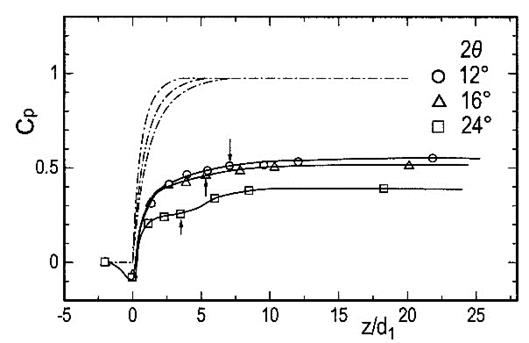Figure 3. Longitudinal distributions of wall static pressure for steady flow at Re =20000. The arrow,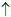: diffuser exits.

In diffusers, for steady flow, the kinetic energy of the flow converts into pressure, and then the static pressure rises in the downstream direction. Figure 3 shows t he distributions of the wall static pressure in the steady flow for the three diffusers. In the figure, the pressures, P, are normalized by the dynamic pressure, ρWa 1 2 /2, in the upstream tube, and it is expressed in the form of the pressure coefficient, Cp, which is defined as Cp=(P-Pref)/( ρWa 1 2 /2), with ρ being the density of the fluid. The positions indicated by arrows show the exit of each diffuser. Moreover, the dash-dotted lines denote the pressure distributions in the axial direction theoretically obtained using the Bernoulli’s theorem. When the angle of divergence becomes large, flow separation occurs, resulting in the wall static pressure taking a considerably lower value than the theoretical value. The pressure in each diffuser is recovered in the downstream straight tube.

Although a value of z/d1 at which the pressure distribution shows a peak is not apparent, it can be considered that the pressure has almost ceased to rise at the station, 5d1, downstream from the exit of each diffuser, i.e., at z= +5d1.

On the other hand, for the pulsating flow with a periodic change in the flow rate, the drop and rise of the pressure in the longitudinal direction are required to accelerate and decelerate the fluid, respectively, with time. Hence, the amplitude of the pressure variation is predicted to be proportional to the pulsation frequency, ω. Consequently, the pressure distribution in the diffuser, varying with time, is complex.

3.1.1. Distribution of Wall Static Pressure and Its Variation with Time

Figure 4 shows an example of the wall static pressure, P, for Tube I with 2θ = 12° for the pulsating flow. Here, Cp is the pressure coefficient and defined as Cp = (P-Pref) / (ρ Wa1,ta2 /2). In the figure, the broken line indicates the time-averaged value, and the chain line indicates the result for a steady flow at the same Reynolds number, Reta. In the upstream tube, Cp changes by the phase leads of approximately 85° against the flow rate variation. The time-averaged value of Cp is slightly larger than one for the steady flow at Re=2000. On the other hand, the pressure in the diffuser rises with an increase of z/d1, except for a part of the period (»300°) when it shows a gentle favorable gradient.

We examine the pressure rise, ΔPL, along the length L, i.e., between the inlet and the exit of the diffuser. The representative result is shown in Fig. 5, in which ΔPL is nondimensionalized by the dynamic pressure based on Wa1,ta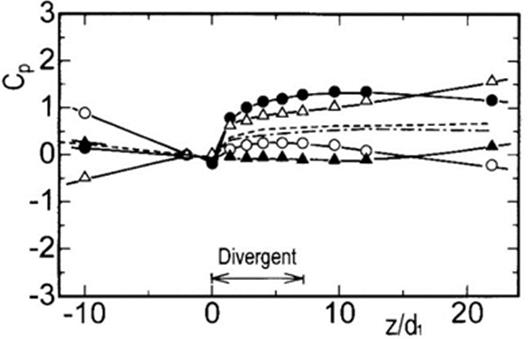Figure 4. Longitudinal distribution of wall static pressure (2θ=12°, α=20, Reta =20000, η= 0.5). :=0°, ●:=90°, :=180°, ▲:=270°, - - -: time-averaged, ¾ - ¾: steady flow (Re=20000).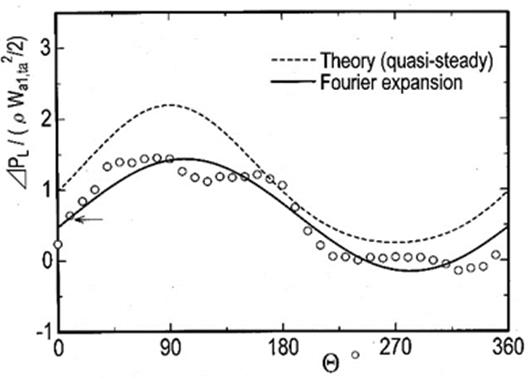Figure 5. Differenc between pressure at the inlet and the exit of the diffuser (2θ=12°, α=20, Reta =20000, η= 0.5). : steady flow (Re=20000).

in the upstream tube. In the figure, the broken line denotes the result that is theoretically obtained using the Bernoulli’s theorem for a quasi-steady flow. It is expressed as

ΔPL,th / (r Wa1,ta2 /2) = (1 +η sin)2(1- m-2).    (2)

Moreover, the symbol, , indicates the pressure rise, ΔPL,s, for the steady flow at Re=20000, with the same cross-sectional averaged velocity, Wa1,ta. The pressure rise, ΔPL, in the pulsating flow changes almost in a sinusoidal manner. However, it gets behind the variation of the flow rate. The phase difference,Φ, between the fundamental waveform of ΔPL and Q becomes large with the increase in the Womersley number. Here, the ΔPL waveform is developed using the Fourier series, denoted by the solid line in Fig. 5. Incidentally, ΦF changes approximately from -5° to -60° when α increases from 10 to 40, as shown later in Fig. 7. On the other hand, ΔPL has a value lower than the theoretical one for the quasi-steady flow. Furthermore, ΔPL takes approximately zero values for the phases, 230~340°, with a small flow rate.

As mentioned previously, for the pulsating flow in the diffusers, the pressure at the exit of the diffuser rises, when the cross-sectional averaged velocity is large; moreover, it is in a decelerative phase. That is, ΔPL becomes large from the latter half of the accelerative phase to the middle of the decelerative phase (»50~180°) as seen in Fig. 5. In contrast, Cp exhibits a small change in the axial direction from the ending of the decelerative phase to the first half of the accelerative phase (» 230~330°). Accordingly, the kinetic energy, which needs to be converted to pressure, is small; hence, to accelerate the fluid in the axial direction, the pressure should be reduced at the downstream. Therefore, it can be understood that the pressure distribution at the beginning of the accelerative phase shows a larger favorable gradient for the higher Womersley number at which the fluid is strongly accelerated in the streamwise direction.

Furthermore, considering practical use, it is desirable to establish a convenient expression for the pressure rise, ΔPL, for the pulsating flow. Hence, we introduce an approximate analysis by assuming the quasi-steady state and considering an unsteady inertia force. In the analysis, we use the one-dimensional equation of unsteady motion. Thus, in the next paragraph, we perform an approximate analysis of ΔPL.

3.1.2. Approximate Analysis Concerning the Pressure Rise ΔPL

The equation of one-dimensional fluid motion is expressed as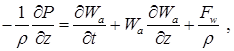(3)

where Wa is the cross-sectional averaged velocity. In the above equation, the third term, Fw, in the right hand side represents the pressure losses in the section of Δz, which are due to the divergence of the tube and the wall friction of the fluid. When Wa is expressed by the flow rate, Q, and the cross-sectional A, i.e.(4)

Eq. (3) is written as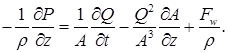(5)

Substituting Eqs. (1) and (4) into Q and A in Eq. (5), respectively, and integrating its equation in the section from z =0 to L, we can obtain the expression of the pressure rise, ΔPL.

For steady flow, the pressure rise, ΔPL,s, is derived as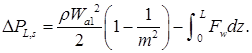(6)

Here, the first term in the right hand side shows the theoretical pressure rise obtained from the Bernoulli’s theorem. Moreover, the second term denotes the pressure drop due to the flow losses, written as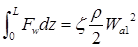(7)

with the pressure loss coefficient, ζ. Thus, the following expression for ΔPL,s is given: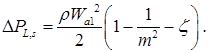(8)

On the other hand, when Eq. (5) is integrated for the pulsating flow, we apply the following relation in the quasi-steady flow to the third term in the right hand side.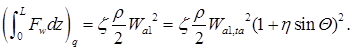(9)

As a result, the expression of ΔPL is obtained as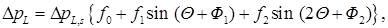(10)

where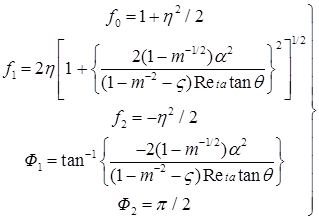(11)

In the above equation, ζ and ΔPL,s, represent the pressure loss coefficient (Eq. (7)) and the pressure rise (Eq. (8)), respectively, in the case of steady flow with the same flow rate as the mean value, Reta, of the pulsating flow.

First, we consider the time-averaged pressure rise, ΔPL,ta. From the approximate analysis,

ΔPL,ta = f0 ΔPL,s. (12)

ΔPL,ta takes a large value, which is f0 times as much as that of the steady flow, being independent of the Womersley number, α. The illustration of the results is omitted because of limited space, and refer to Fig. 4.

Secondly, we discuss the varying components of ΔPL. Furthermore, as clearly seen from Fig. 5 and the approximate expressions of Eqs. (10) and (11), the second or higher-order components are small, approximately η/4 as compared with the first one. Therefore, we examine the amplitude value, ΔPL,os, and the phase difference, Φ, of the first component. For ΔPL,os, we define the coefficient as

k = ΔPL,os /(rWa1,os2/2) . (13)

In the approximate analysis, k is expressed as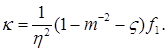(14)

Figure 6 shows the results. They are plotted with the characteristic number of α2/Reta as abscissa against ηk as ordinate, which is taken from the viewpoint that k varies inversely with η. The solid lines indicate the approximate results for Reta = 20000, in which the values measured in the steady flow are used for ζ. The measurement data accurately shows the dependence on the flow parameters as denoted by the approximate analysis. That is, ηk increases almost in proportion to α2/Reta as an unsteady inertia force is intensified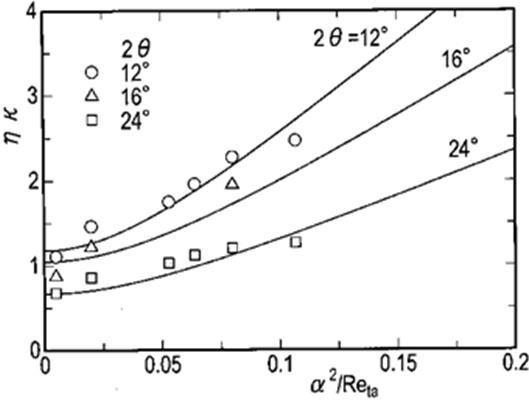Figure 6. Relationship between ηk and α2/Reta. Lines denote the results of Eq. (14).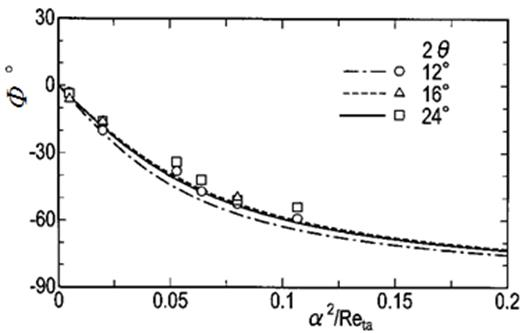Figure 7. Relationship between Φ and α2/Reta. Lines denote the results of Eq. (11).

with an increase in a. Furthermore, ΔPL,os, which is required for the deceleration and the acceleration of the flow, proportionately increases with L, as the fluid mass involved in the divergent section multiplies with the smaller 2θ and the longer, L.

Next we consider the phase difference Φ between ΔPL,os and the flow rate variation. The experimental results are given in Fig. 7. Each line in the figure denotes the approximate expression Φ1 of Eq. (11) for Reta = 20000. According to the approximate results, Φ at 2θ =12° is a little more negative than one for another. Nevertheless, the experimental results show that the divergence angle 2θ has little effect on Φ. Consequently, the phase lag becomes large with an increase of α2/Reta, and it changes almost according to the expression Eq. (11).

3.2. Velocity Characteristics

In this section, we extract a feature of the phase-averaged velocity characteristics of the pulsating flow in the diffusers from the results obtained by the I-type hot-wire probe. In the discussion, observations obtained by a smoke wire method and by the solid tracer method using water are used for reference data. In the hot-wire measurement with the I-type probe, the measurement accuracy deteriorates in the following cases: 1) in the region from the outer edge of the flow into the conical diffuser to the neighborhood of the wall; 2) the flow at a position and time when the ratio of the radial component of the velocity to the axial component is large. This is because it is hard to distinguish the flow direction in such cases, as described in section 2.2.

3.2.1. Changes in Centerline Velocity with Time

Initially, we will explain the outline of the flow features by focusing on the velocity along the diffuser axis. Figure 8 shows the changes in the phase-averaged velocity along the diffuser axis, Wc, with time at the exits of the diffusers. The centerline velocity, Wc, at z/d1= -2 in the upstream straight tube changes in a sinusoidal manner with a phase lag of approximately 5° from the flow rate variation. However, the flow in the diffuser extends further towards the wall as 2θ decreases. Consequently, Wc at the diffuser exit is decreased, and the phase lag of the Wc waveform relative to the flow rate variation is increased slightly. The phase lags obtained from the fundamental component derived from the expansion of the Fourier series are approximately 35° and 20° for 2θ=12° and 24°, respectively. In addition, the phase lags correspond to the time required for the fluid flowing into the diffuser at the maximum flow rate to reach the diffuser exit.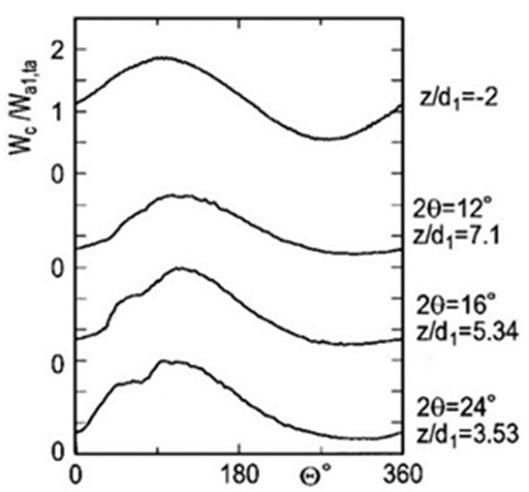Figure 8. Waveforms of phase-averaged velocity Wc on the diffuser axis at diffuser exits (α =20, Reta =20000, η = 0.5).

Moreover, as z/d1 increases, the flow state in each diffuser is divided into two parts of a period with a large Wc and another period. This can be seen from the change in the turbulence intensity. Therefore, the sinusoidal Wc waveform becomes distorted. To examine the distortion, we introduce the velocity ratio, ε, given by the following equation:

ε = (Wc,max - Wc,min) / (2Wc,ta),           (15)

where subscripts max and min indicate the maximum and minimum values in a cycle, respectively. The obtained results are shown in Fig. 9, in which each symbol ¯ denotes the position of a diffuser exit. The ratio, ε, at α=20 is larger than that at α=10. For the diffusers with 2θ=16° and 24°, the distortion is largest near the exit.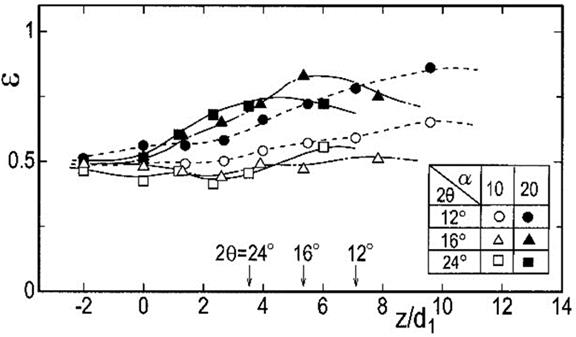Figure 9. Distribution of velocity ratio ε along the diffuser axis z/d1 (Reta =20000, η = 0.5).

3.2.2. Distributions of Phase-Averaged Velocity

Figures 10 to 13 show distributions of W, which is normalized by the time and cross-sectional-averaged velocities in the upstream tube, Wa1,ta, for Reta=20000 and η=0.5. Illustrations are given for four representative phases in a pulsation cycle.

When the flow enters the diffuser, the main current entrains the surrounding fluid at its boundary, and the shear layer there develops into massive ring-shaped vortices. At that time, a strong adverse pressure gradient appears on the diffuser wall immediately behind the inlet. Thus, the fluid near the wall in the vicinity of the inlet corner is forced to flow towards the boundary of the main current. The massive vortices, which rotate downstream from the inlet, move in the radial direction owing to the pushing of the fluid in the accelerative phase. Consequently, the radial position with the maximum velocity shifts from the diffuser axis towards the wall as z/d1 increases. Such a state can be seen in the flows with α=10 for the diffusers with 2θ=16° and 24°, the distributions of which are shown in Figs. 10 and 11. In the figures, the above-mentioned velocity distributions can be recognized at a phase angle of»90° with a large flow rate after the acceleration phase. It is interesting that a characteristic distribution appears in the pulsating flow at a rather low Womersley number in the above diffusers with large divergence angles of 2θ=16° and 24°.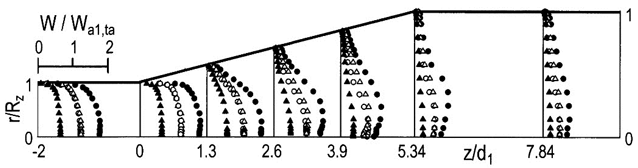Figure 10. Distributions of W (2θ =16°, α =10, Reta =20000, η = 0.5). :=0°, ●:=90°, :=180°, ▲:=270°.Figure 11. Distributions of W (2θ =24°, α =10, Reta =20000, η = 0.5). :=0°, ●:=90°, :=180°, ▲:=270°.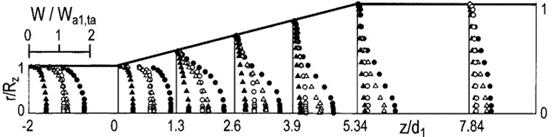Figure 12. Distributions of W (2θ =16°, α =20, Reta =20000, η = 0.5). :=0°, ●:=90°, :=180°, ▲:=270°.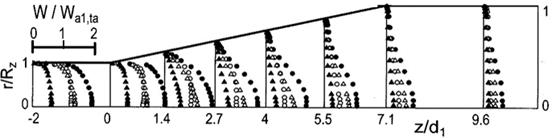Figure 13. Distributions of W (2θ =12°, α =20, Reta =20000, η = 0.5). :=0°, ●:=90°, :=180°, ▲:=270°.

On the other hand, for the case of α=20, as shown in Fig. 12, the period of the variation of the flow rate is reduced to a quarter of that for α=10. Thus, even if the fluid flows in the acceleration state into the diffuser, there is insufficient time for the shear layer of the main current to develop into massive vortices. Meanwhile, the phase advances into the decelerative phase in which the flow rate decreases. Therefore, the radial position of the maximum value of W still remains on the diffuser axis throughout the cycle. On the other hand, the phase difference between the varying component of the local velocity and the flow rate becomes larger in the cross section. Hence, the W distributions in the accelerative and decelerative phases differ noticeably in shape. To consider the case of phases with the same instantaneous flow rate, we compare the distribution at=0° with that at=180°. In the former, as the pressure does not change significantly in the streamwise direction, the distribution is flat in the central part of the cross section. In contrast, when=180°, at which the pressure increases with z/d1, the velocity decreases near the wall and so the distribution develops a protruding shape.

For the diffuser with a small divergence angle of 2θ=12°, the W distribution with α=10 changes with time along the diffuser axis, similar to case in the steady flow (not shown owing to limited space). However, for α=20, the velocity distribution develops a protruding shape with increasingfor»0-270°, as seen in Fig. 13.

3.2.3. Estimate of Backward Flow Rate

In Figs. 10 to 12, a backward flow is observed near the wall, depending on the phase. In such a case, the flow rate obtained by integrating the velocity distributions measured using the I-type probe becomes more excessive than that suggested using Eq. (1). However, using the two values, we can approximately estimate the backward flow rate.

Figure 14 shows an example of the variation of the backward flow rate, q, along the diffuser axis for 2θ=16°. For α=10, q is large in the first stage of the decelerative phase. At the station of z/d1=3.9 near the diffuser exit, the backward flow rate appears to be 30% of the instantaneous flow rate, Q. This flow in the negative direction is attributed to the massive vortices at the outer edge of the main current approaching the wall and due to a strong adverse pressure gradient. On the other hand, q for α=20 is half of that for α=10. Therefore, a decrease in q reduces the energy loss, and this causes ΔPL,ta to increase.

The reduction of the backward flow rate at the diffuser exit implies that the uniformity of the velocity distribution is high. The result wherein the flow uniformity increases with the pulsation frequency was also obtained in the experiment conducted by Benjamin et al. , which was carried out at reasonably high frequencies for a 60° conical diffuser. Consequently, the time-averaged pressure rise increases, and the pressure loss decreases as the pulsation frequency increases.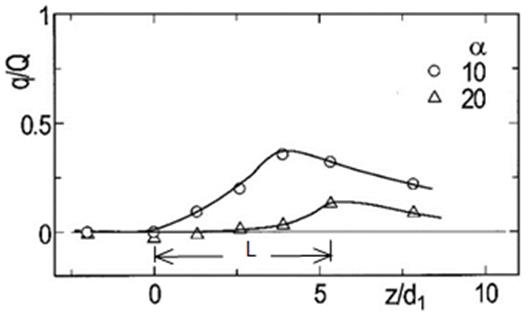Figure 14. Backward flow rate relative to given axial flow rate (2θ =16°, Reta =20000, η= 0.5,= 90°).

4. Conclusions

The characteristics of the conical diffusers with divergence angles of 2θ=12°, 16°, and 24° were investigated experimentally for pulsating turbulent flows. The effects of the nondimensional flow parameters and the divergence angle on the flow field have been examined. The principal findings of this study are summarized as follows:

(1)   The approximate expressions for the pressure rise, ΔpL, between the inlet and the exit of the diffuser are in good agreement with the experimental results. These are practically very useful.

(2)   The time-mean pressure rise is larger than that in the steady flow, increasing in proportion to the flow rate ratio, η. The amplitude of, ΔpL, is larger for smaller divergence angles. Its value and the phase lag from the flow rate depends and increases with the characteristic number, α2/Reta.

(3)   The distribution of the pressure coefficient, Cp, along the tube axis is high in the phase from the latter half of the acceleration to the middle of the deceleration. On the other hand, it is low in the rest of the phases.

(4)   The sinusoidal change in the phase-averaged velocity with time becomes distorted as the fluid proceeds in the diffuser, and its degree increases with an increase in α and 2θ. Thereby the distributions of W vary in a highly complicated manner with time. In addition, lower the value of α and larger the value of 2θ, higher will be the backward flow rate.

(5)   For the flow with α=10 in the diffusers with large divergence angles of 2θ=16° and 24°, the radial position with the maximum velocity shifts towards the wall for the phases with a large flow rate, and the W distribution is depressed on the diffuser axis. On the other hand, for the flow at α=20, W takes a maximum value on the diffuser axis throughout the cycle and shows a profile swelling in the central part of the cross section when the flow rate increases.

Acknowledgements

The author would like to thank Mr. J. Morita and Mr. A. Ohnishi for their assistance with the experiments during their time as graduate students.

Nomenclature

Cp: pressure coefficient =(P-Pref)/(ρWa1,ta2/2)

d1, d2: diameters at the inlet and exit of the diffuser, respectively

L: diffuser axial length

m: area ratio

p: instantaneous pressure

P: phase-averaged pressure

q: backward flow rate

Q: flow rate

r, z: coordinate system

Rz: distance between the diffuser axis and the wall

Reos: oscillatory Reynolds number = Wa1,os d1 /ν

Reta: mean Reynolds number =Wa1,ta d1 /ν

t: time

w: instantaneous axial velocity

w’: turbulence intensity

W: phase-averaged axial velocity

α: Womersley number (nondimensional frequency) = (d1/2) (w/ν)1/2

ΔPL: pressure rise between L

ε: velocity ratio =(Wc,max-Wc,min)/(2Wc,ta)

ζ: pressure loss coefficient

η: flow rate ratio = Wa1,os /Wa1,ta

2θ: divergence angle: phase angle = ωt

k: nondimensional amplitude of the pressure rise

ν, ρ: kinematic viscosity and density of fluid

ω: angular frequency

Φ: phase difference

Subscripts

a: cross-sectional averaged value

c: value on the diffuser axis

max, min: maximum and minimum values, respectively

q, s: quasi-steady and steady flows, respectively

ref: reference quantity at z/d1= -2

ta, os: time mean and amplitude values

th: ideal value

1, 2: values in the upstream and downstream tubes, respectively

References

1. Kline, S. J., Abbott, D. E. and Fox, R. W. (1959). Optimum design of straight-walled diffusers, Journal of Basic Engineering, Transactions of ASME, Series D, Vol. 81, pp. 321-329.
2. Japan Society of Mechanical Engineers ed. (1980). JSME Data Book: Hydraulic losses in pipes and ducts, pp. 56-62, The Japan Society of Mechanical Engineers (in Japanese).
3. Mizuno, A. and Ohashi, H. (1984). A study of flow in a two-dimensional diffuser with an oscillating plate, Transactions of the Japan Society of Mechanical Engineers, Series B, Vol. 50, No. 453, pp. 1223-1230 (in Japanese).
4. Mochizuki, O., Kiya, M., Shima, Y. and Saito, T. (1997). Response of separating flow in a diffuser to unsteady disturbances, Transactions of the Japan Society of Mechanical Engineers, Series B, Vol. 63, No. 605, pp. 54-61 (in Japanese).
5. Mochizuki, O., Ishikawa, H., Miura, N., Sasuga, N. and Kiya, M. (2001). Precursor of separation, Transactions of the Japan Society of Mechanical Engineers, Series B, Vol. 67, No. 661, pp. 2226-2233 (in Japanese).
6. Yokota, S., Nakano, K. and Tanaka, Y. (1986). Oscillatory flow in the jet flow region through cylindrical chokes, 1st report: Flow visualization, Journal of the Japan Hydraulics & Pneumatics Society, Vol. 17, No. 6, pp. 469-475 (in Japanese).
7. Iguchi, M., Yamazaki, H., Yamada, E. and Morita, Z. (1990). Velocity and turbulence intensity in a pulsating jet through a sudden expansion, Transactions of the Japan Society of Mechanical Engineers, Series B, Vol. 56, No. 526, pp. 1659-1664 (in Japanese).
8. Benjamin, S. F., Roberts, C. A. and Wollin, J. (2002). A study of pulsating flow in automotive catalyst systems, Experiments in Fluids, Vol. 33, pp. 629-639.
9. King, C. V. and Smith, B. L. (2011). Oscillating flow in a 2-D diffuser, Experiments in Fluids, Vol. 51, pp. 1577-1590.
10. Mat Yamin, A. K., Benjamin, S. F. and Roberts, C. A. (2013). Pulsating flow in a planar diffuser upstream of automotive catalyst monoliths, International Journal of Heat and Fluid Flow, Vol. 40, pp. 43-53.
11. Nabavi, M. (2009). Steady and unsteady flow analysis in microdiffusers and micropumps: a critical review, Microfluidics and Nanofluidics, Vol. 7, pp. 599-619.
12. Hwang, I. -H., Lee, S. -K., Shin, S. -M., Lee, Y. -G. and Lee,J. -Y. (2008). Flow characterization of valveless micropump using driving equivalent moment: theory and experiments, Microfluidics and Nanofluidics, Vol. 5, pp. 795-807.
13. Sun, C. -L., Tsang, S. and Huang, H. -Y. (2015). An analytical model for flow rectification of a microdiffuser driven by an oscillating source, Microfluidics and Nanofluidics, Vol. 18, pp. 979-993.
14. Wang, Y. -C., Lin, S. -H. and Jang, D. (2010). Unsteady analysis of the flow rectification performance of conical diffuser valves for valveless micropump applications, Journal of Mechanics, Vol. 26, Issue 3, pp. 299-307.
15. Wang, Y. -C., Chen, H. -Y. and Hsiao, Y. -Y. (2011). Experimental study of the flow rectification performance of conical diffuser valves, Acta Mechanica, Vol. 219, pp. 15-27.
16. Erath, B. D. and Plesniak, M. W. (2006). An investigation of bimodal jet trajectory in flow through scaled models of the human vocal tract, Experiments in Fluids, Vol. 40, pp. 683-696.
17. Erath, B. D. and Plesniak, M. W. (2010). An investigation of asymmetric flow features in a scaled-up driven model of the human vocal folds, Experiments in Fluids, Vol. 49, pp. 131-146.
18. Sumida, M. (2009). Experimental study of pulsating turbulent flow through a divergent tube, in Matos, D. and Valerio, C. ed., Fluid Mechanics and Pipe Flow, Chapter 11, Nova Science Pub.
19. Nabavi, M. and Siddiqui, K. (2010). A critical review on advanced velocity measurement techniques in pulsating flows, Measurement Science and Technology, Vol. 21, 042002 (19pp).
20. Ohmi, M. and Iguchi, M. (1980). Flow pattern and frictional losses in pulsating pipe flow, Part 3: General representation of turbulent flow pattern, Bulletin of the Japan Society of Mechanical Engineers, Vol. 23, No. 186, pp. 2029-2036.
21. Iguchi, M., Ohmi, M. and Tanaka, S. (1985). Experimental study of turbulence in a pulsatile pipe flow, Bulletin of the Japan Society of Mechanical Engineers, Vol. 28, No. 246, pp. 2915-2922.
22. Sohn, H. C., Lee, H. N. and Park, G. M. (2007). A study of the flow characteristics of developing turbulent pulsating flows in a curved duct, Journal of Mechanical Science and Technology, Vol. 21, pp. 2229-2236.
23. Sumida, M. and Senoo, T. (2015). Experimental investigation on pulsating flow in a bend, Journal of Fluid Flow, Heat and Mass Transfer, Vol. 2, pp. 26-33.

 Contents 1. 2. 2.1. 2.2. 2.3. 3. 3.1. 3.2. 4.
Article ToolsAbstractPDF(4469K)